Скачать презентацию Basic Valuation Basic Valuation Key Terms

a2141096c8b196912607ef54ff15628b.ppt

• Количество слайдов: 48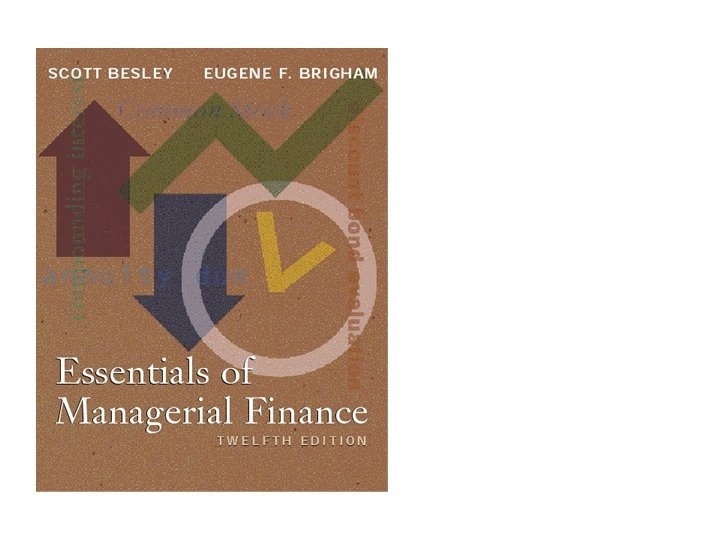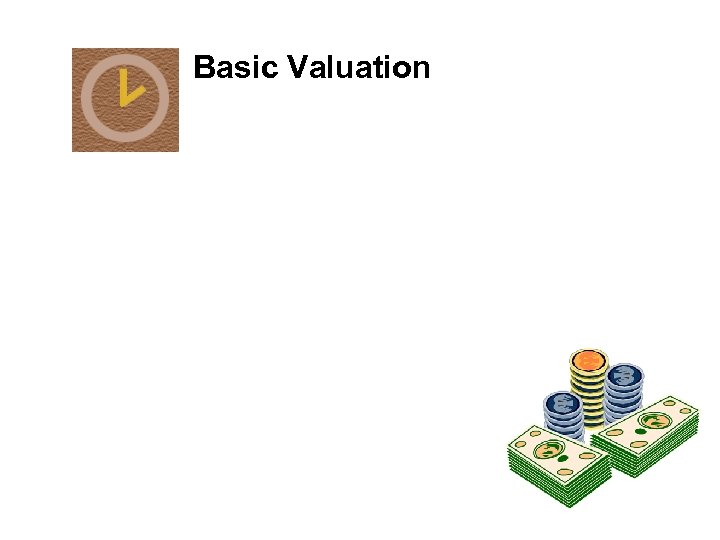Basic Valuation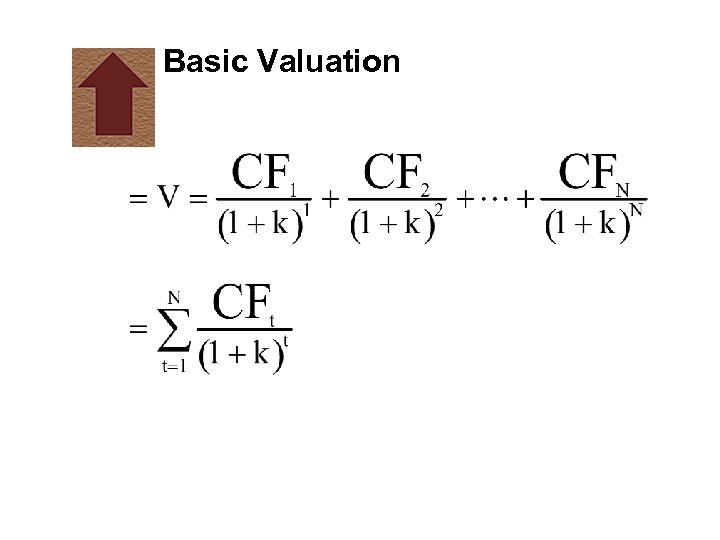Basic ValuationKey TermsKey Terms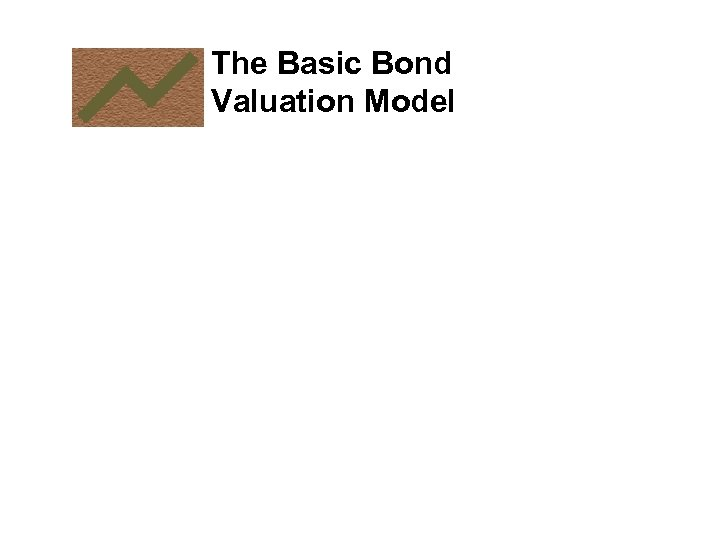The Basic Bond Valuation Model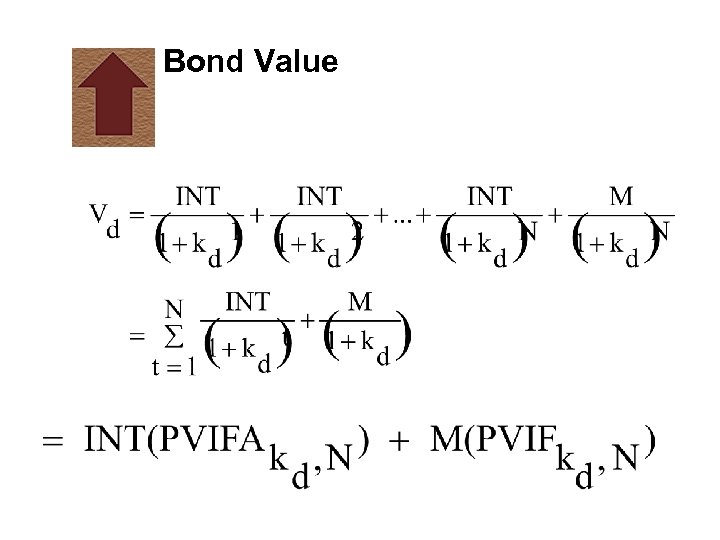Bond Value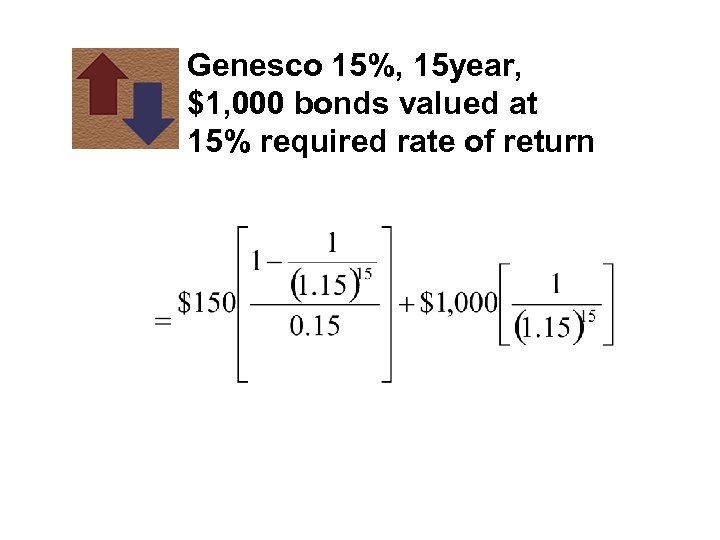Genesco 15%, 15 year, \$1, 000 bonds valued at 15% required rate of return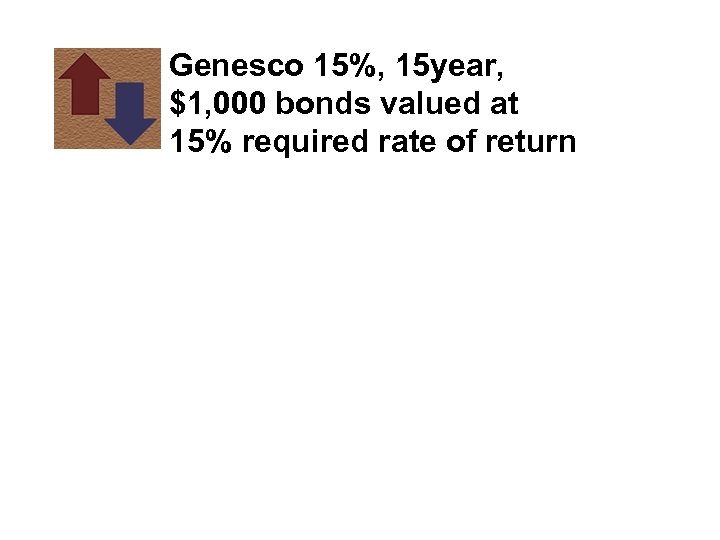Genesco 15%, 15 year, \$1, 000 bonds valued at 15% required rate of return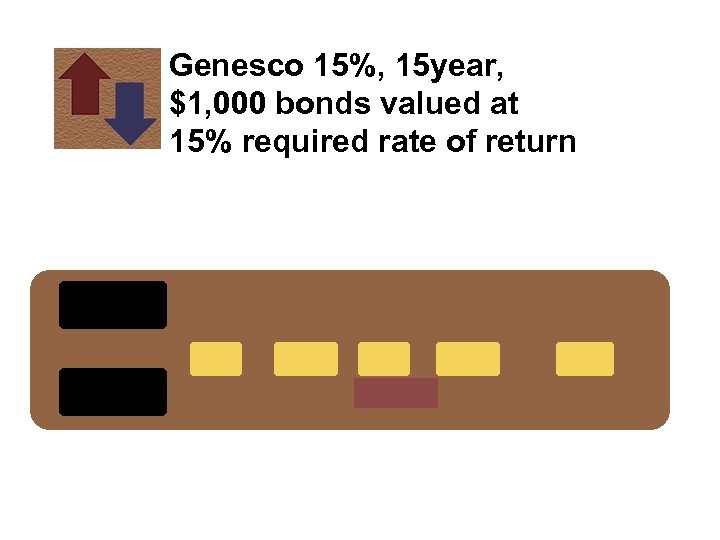Genesco 15%, 15 year, \$1, 000 bonds valued at 15% required rate of return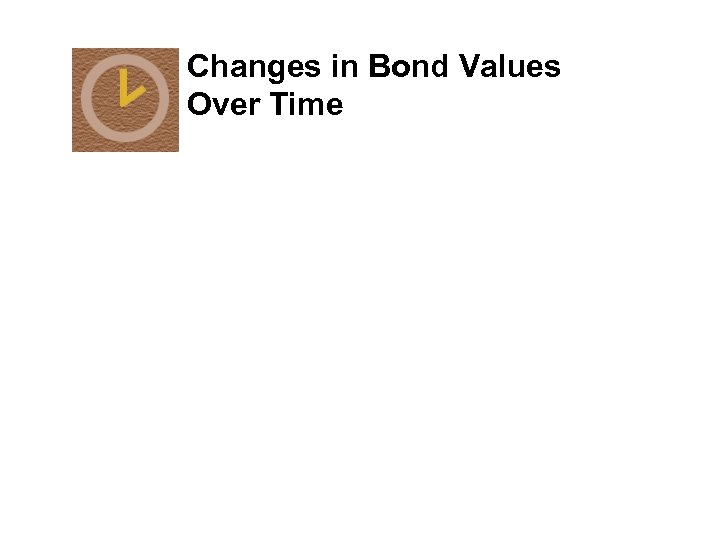Changes in Bond Values Over Time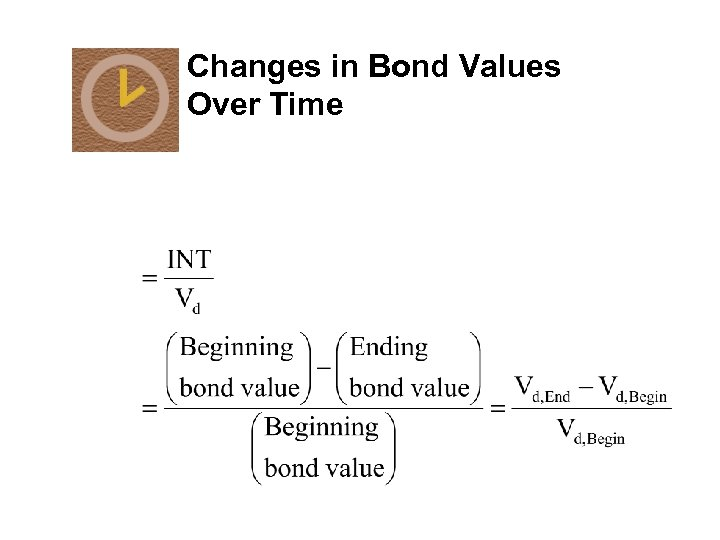Changes in Bond Values Over TimeChanges in Bond Values Over TimeChanges in Bond Values Over Time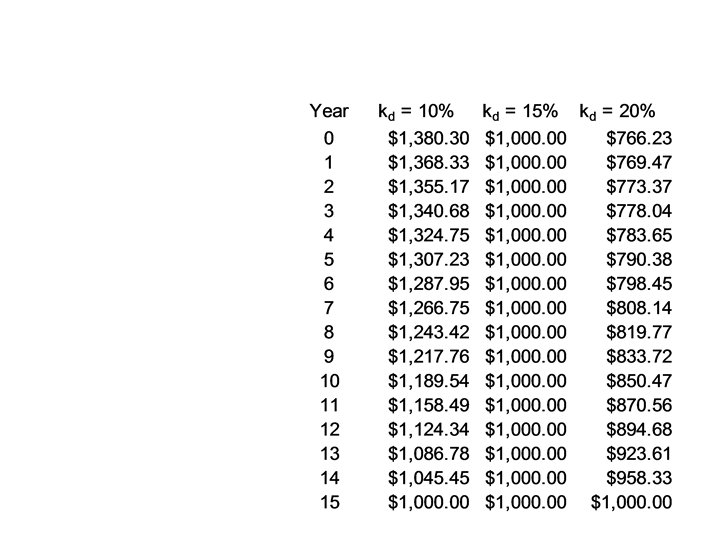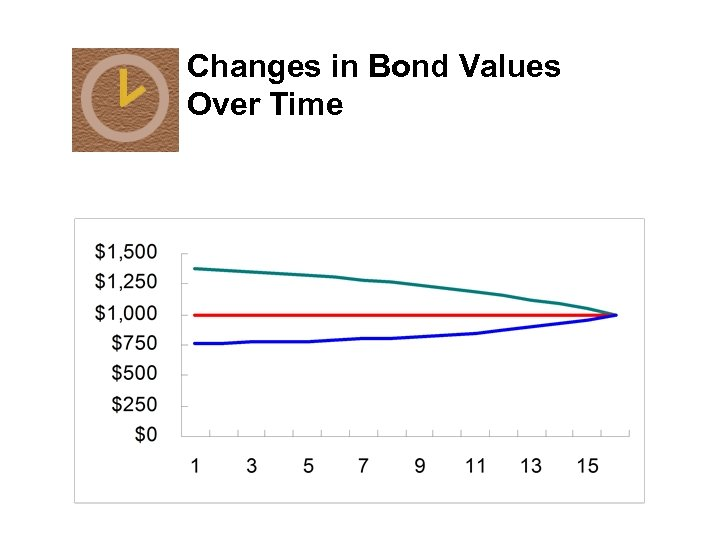Changes in Bond Values Over Time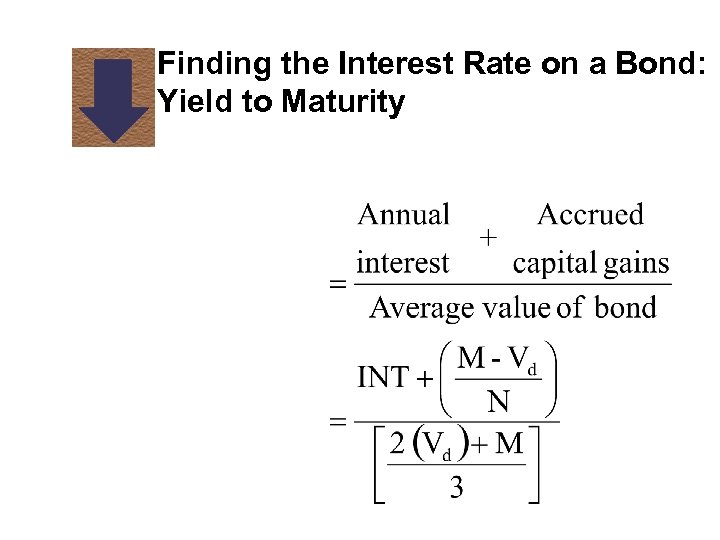Finding the Interest Rate on a Bond: Yield to Maturity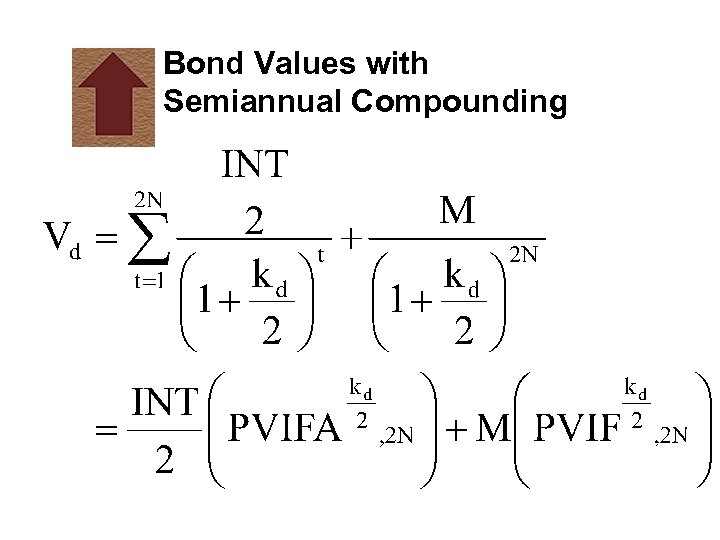Bond Values with Semiannual Compounding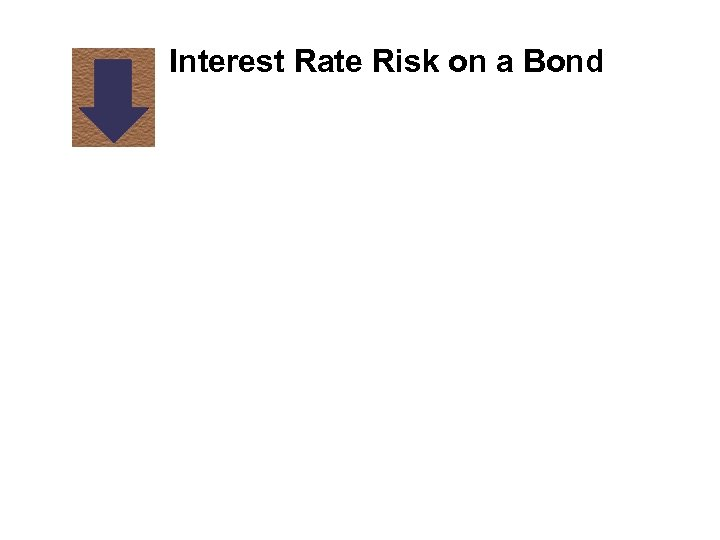Interest Rate Risk on a Bond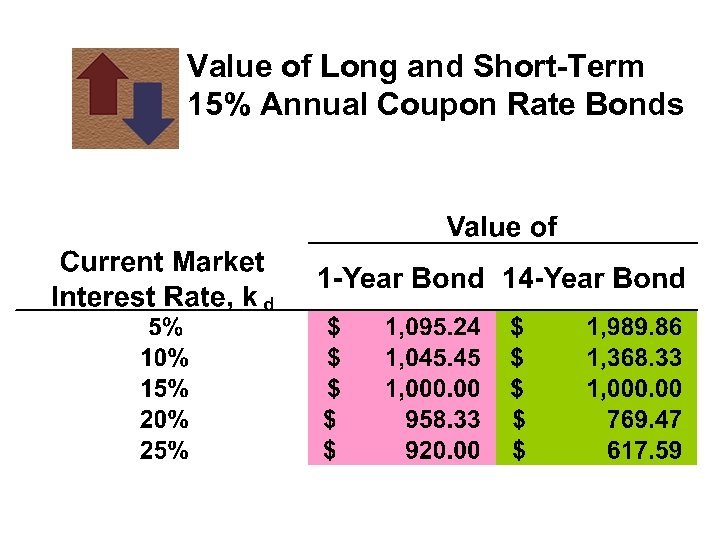Value of Long and Short-Term 15% Annual Coupon Rate Bonds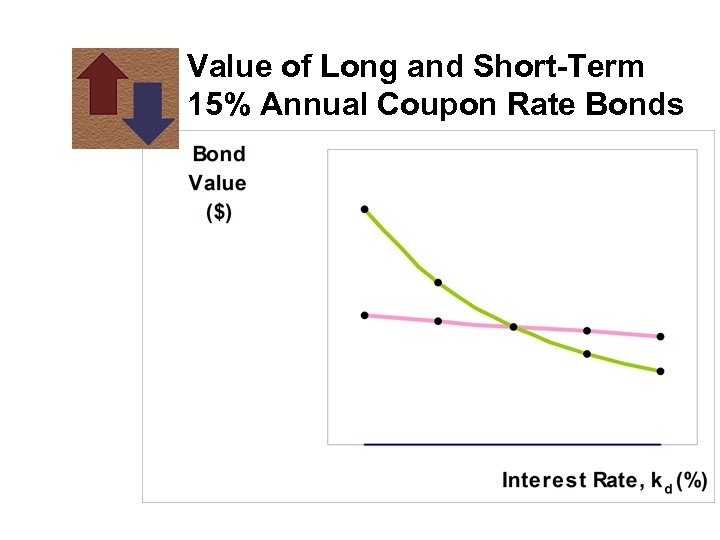Value of Long and Short-Term 15% Annual Coupon Rate Bonds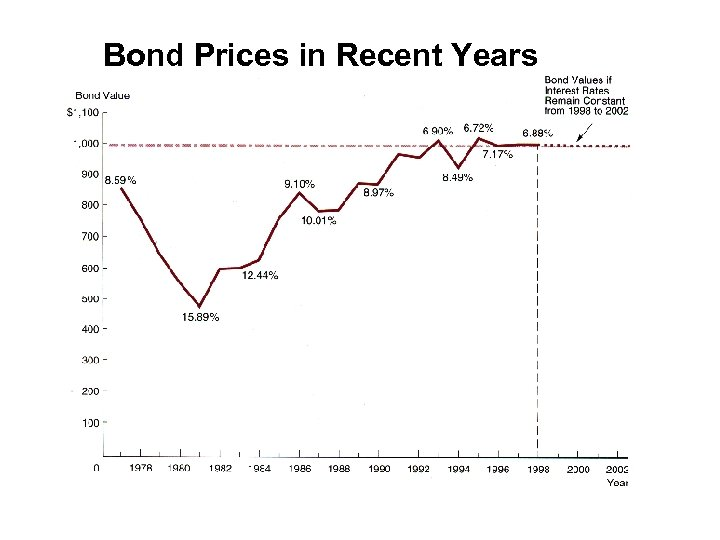Bond Prices in Recent Years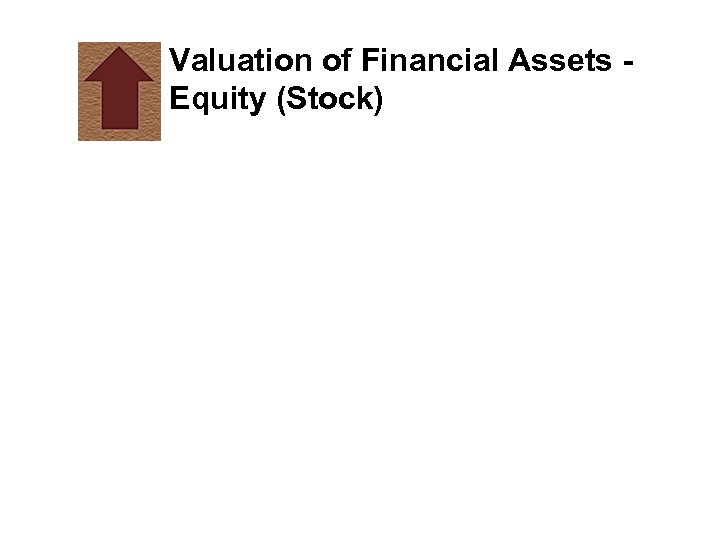Valuation of Financial Assets Equity (Stock)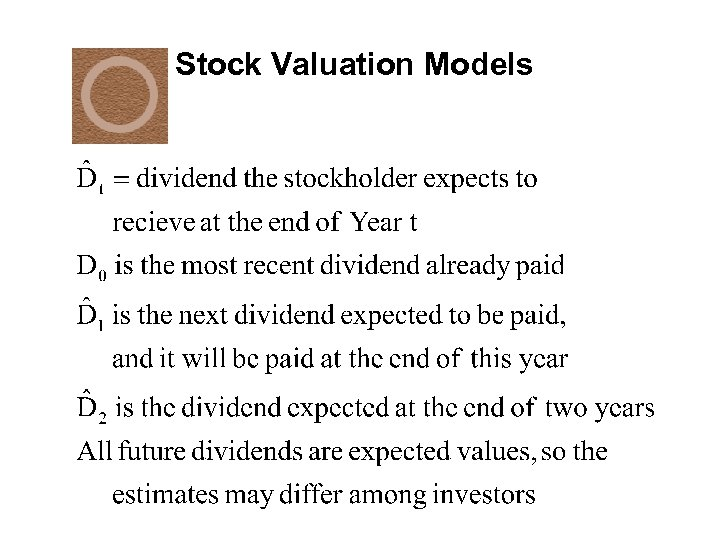Stock Valuation Models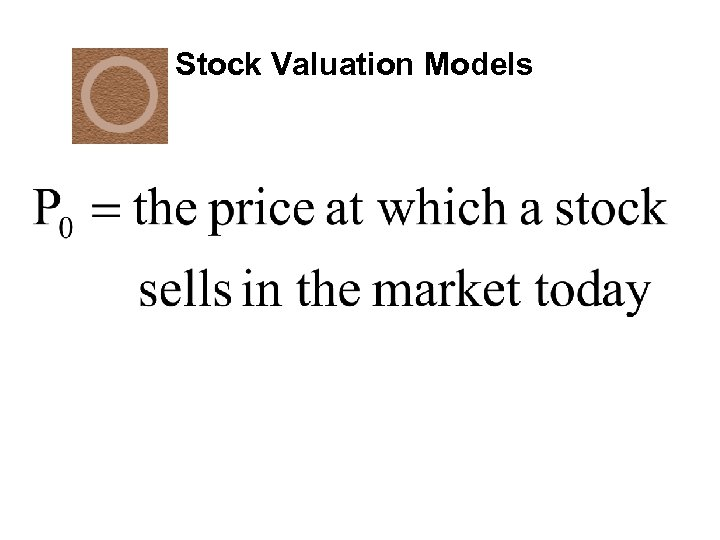Stock Valuation Models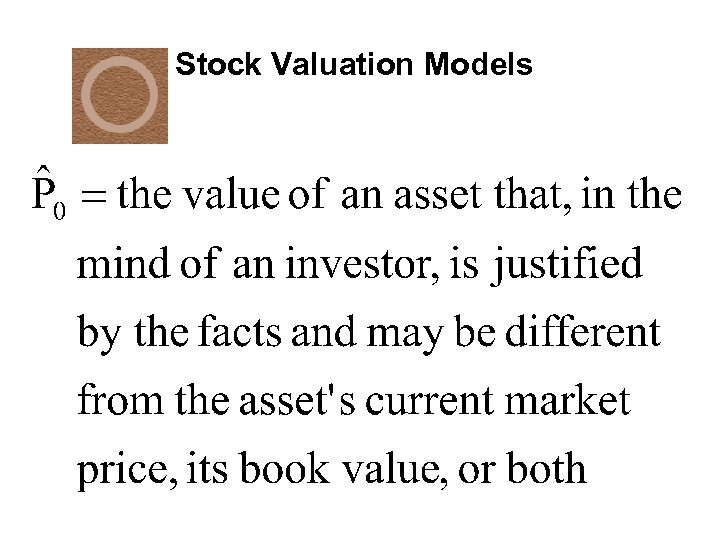Stock Valuation Models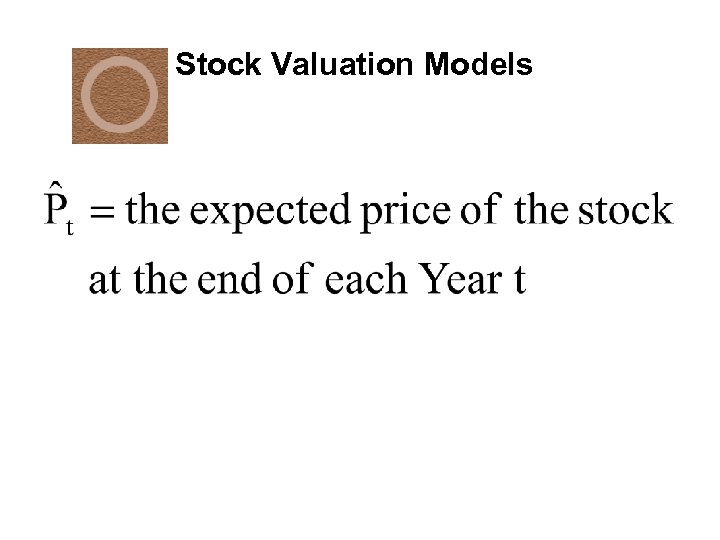Stock Valuation Models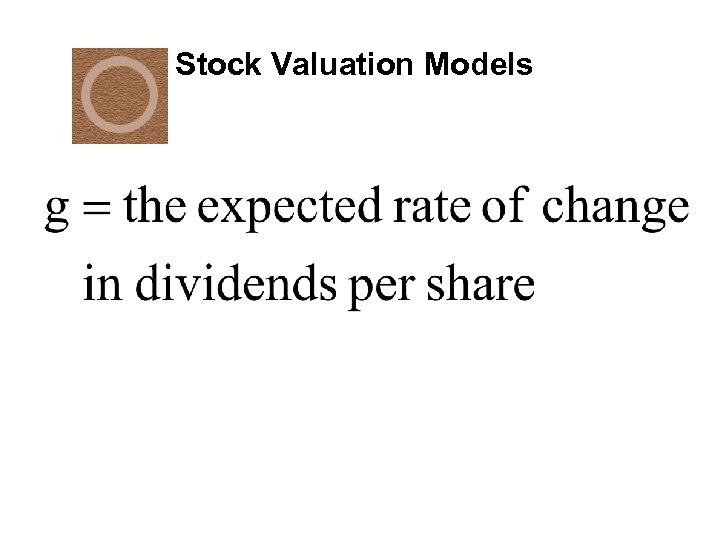Stock Valuation Models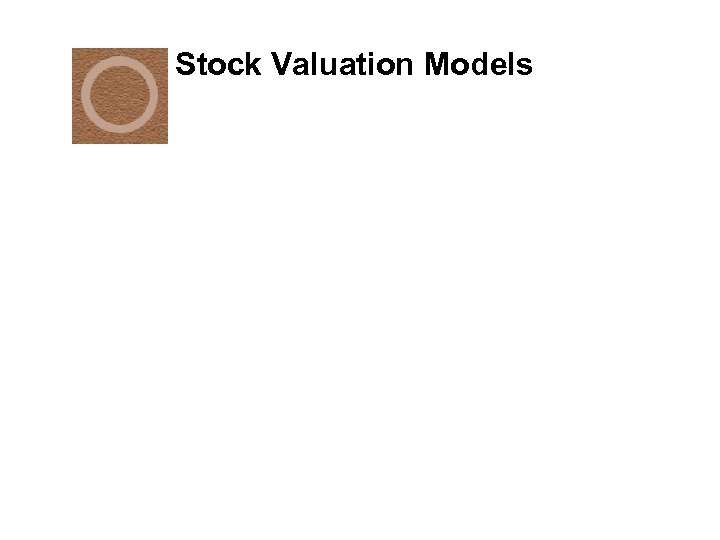Stock Valuation Models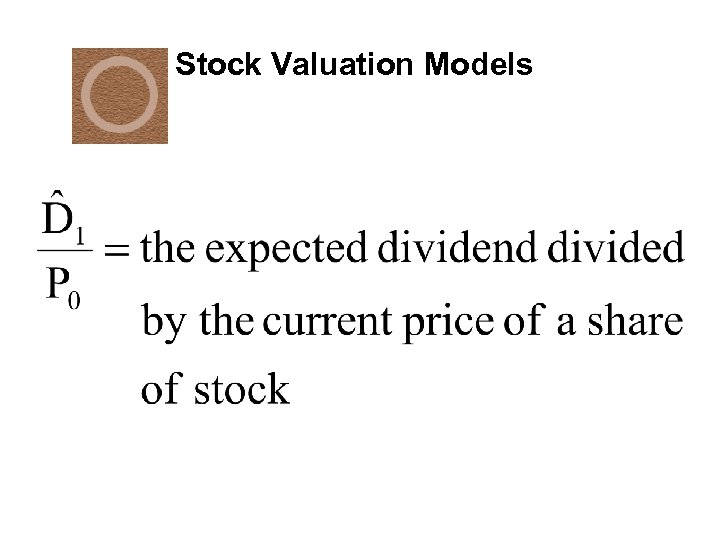Stock Valuation Models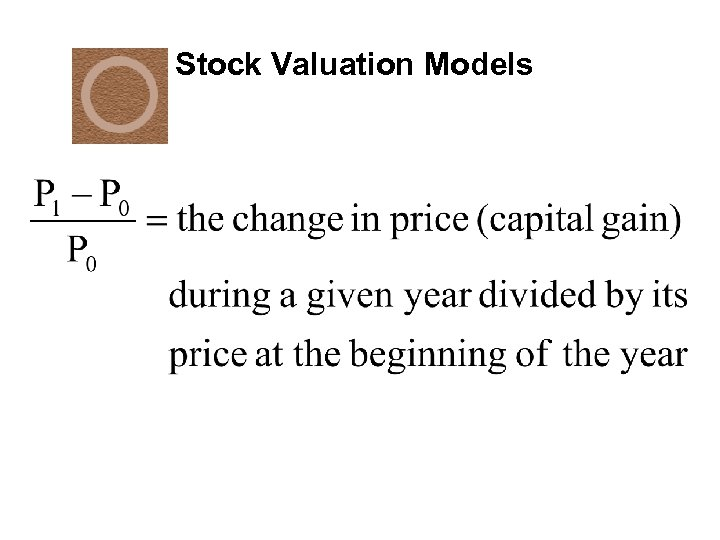Stock Valuation Models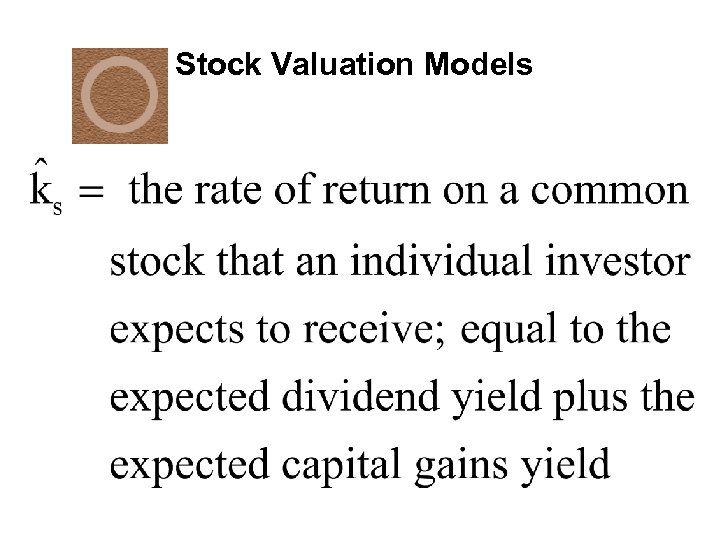Stock Valuation Models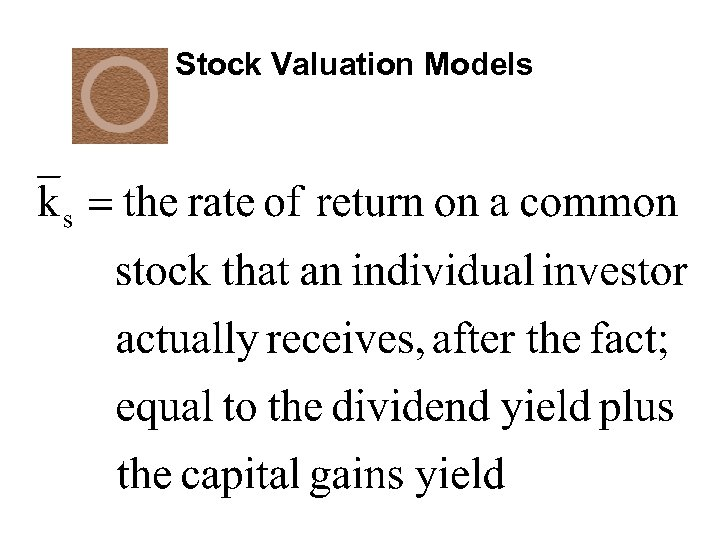Stock Valuation Models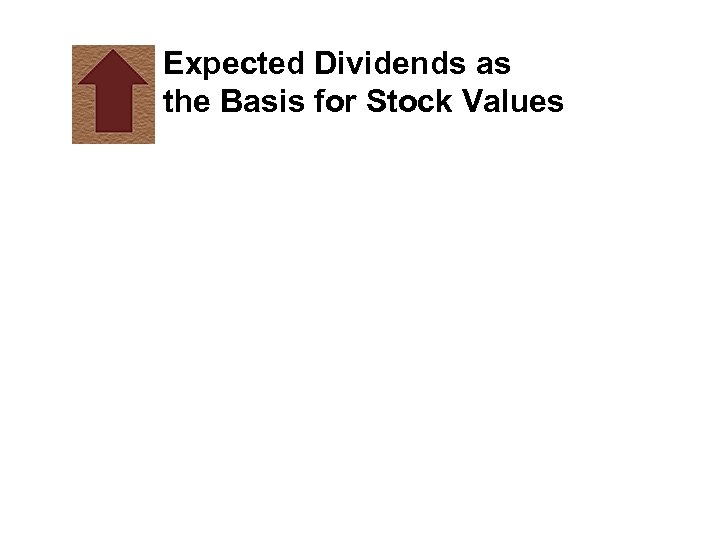Expected Dividends as the Basis for Stock Values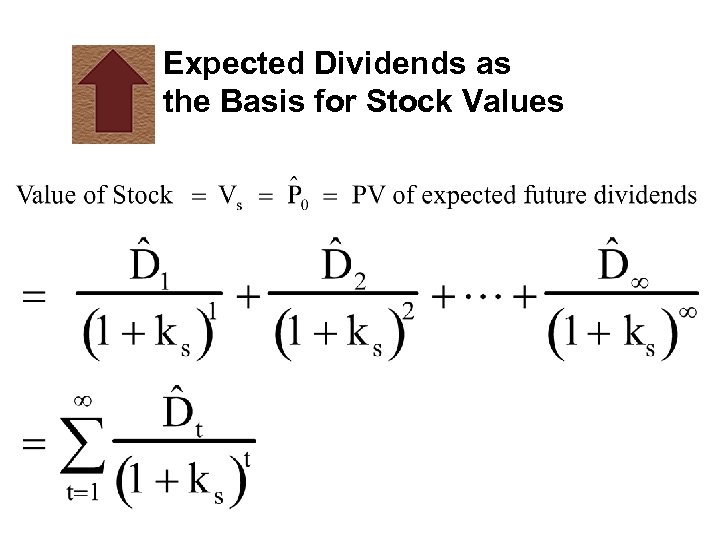Expected Dividends as the Basis for Stock Values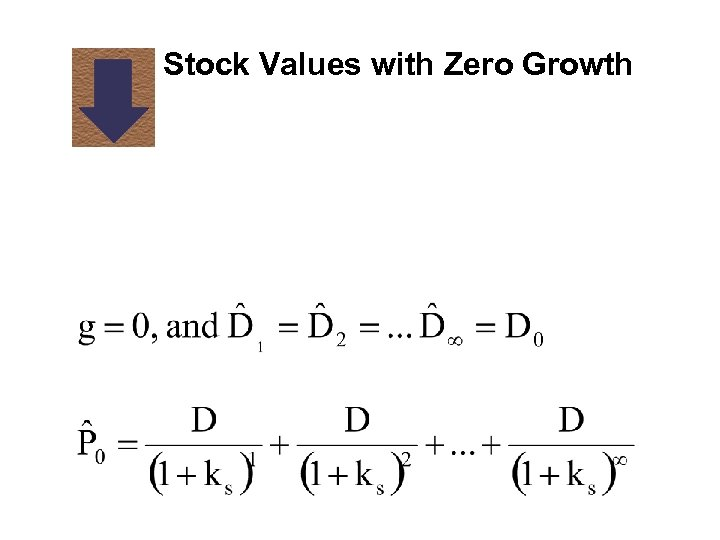Stock Values with Zero Growth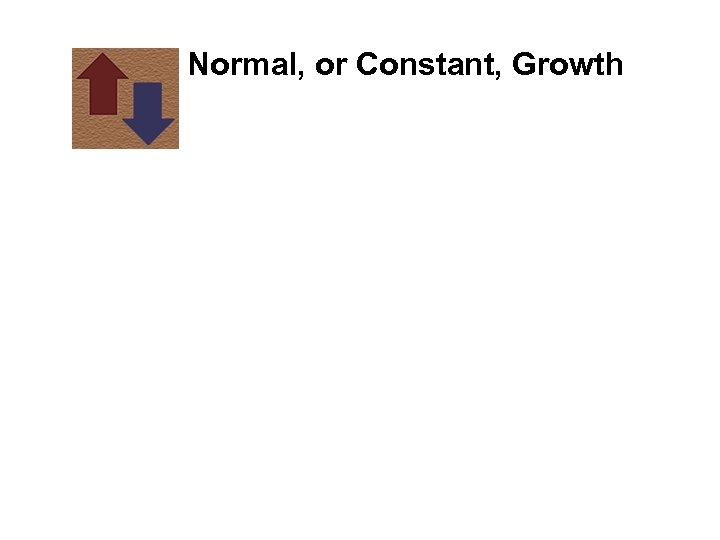Normal, or Constant, Growth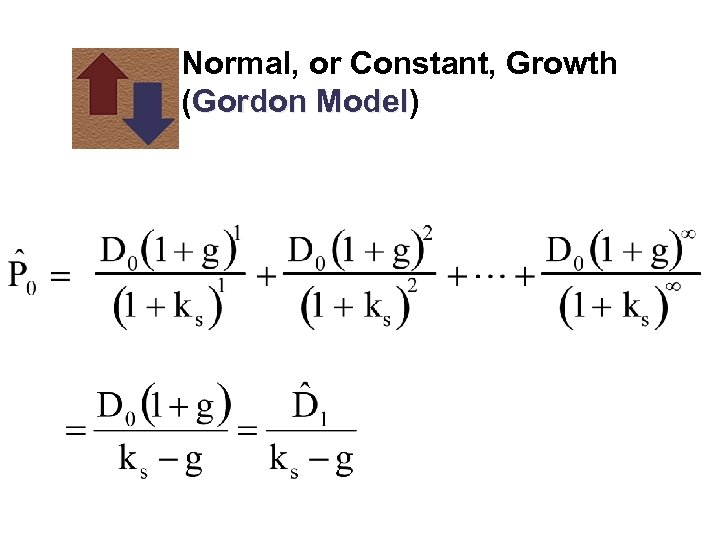Normal, or Constant, Growth (Gordon Model) Model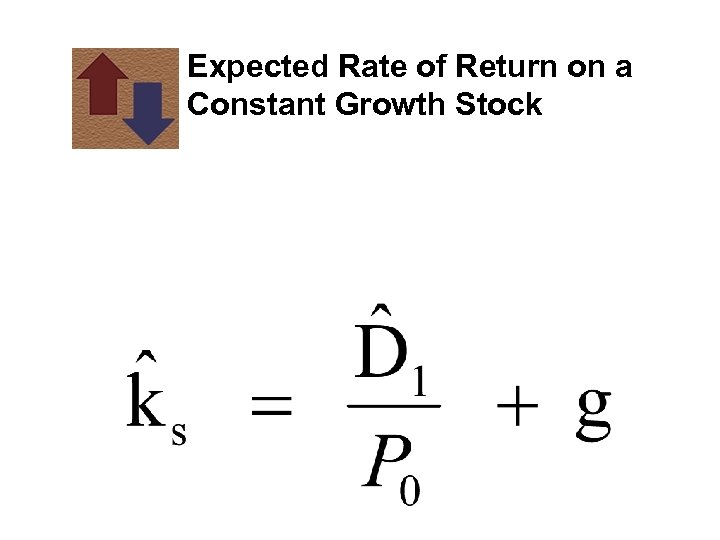Expected Rate of Return on a Constant Growth Stock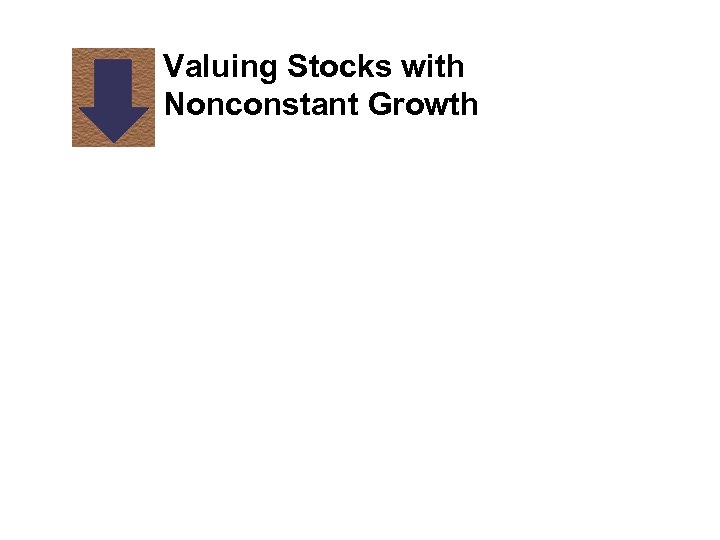Valuing Stocks with Nonconstant Growth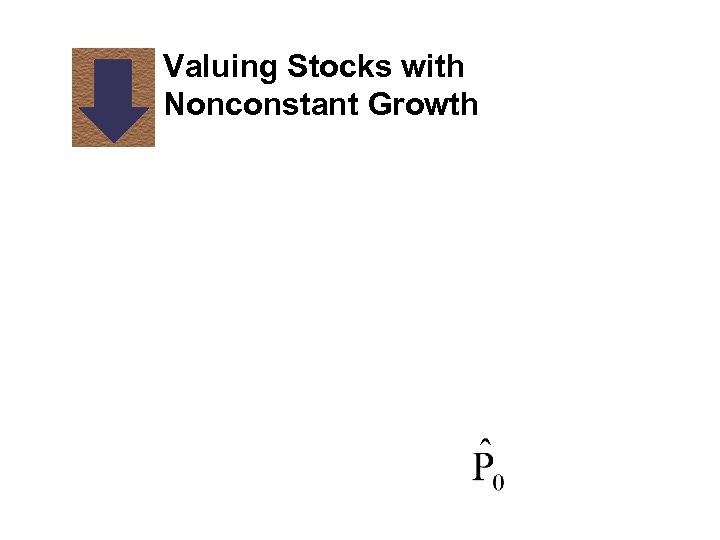Valuing Stocks with Nonconstant Growth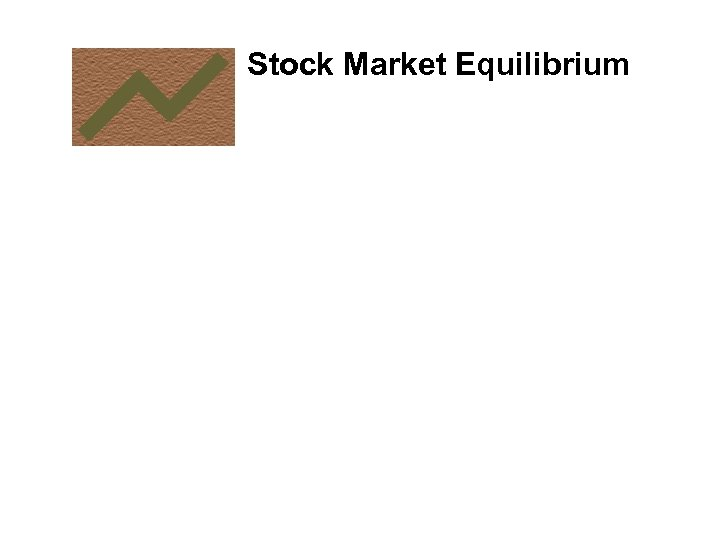Stock Market EquilibriumChanges in Stock Prices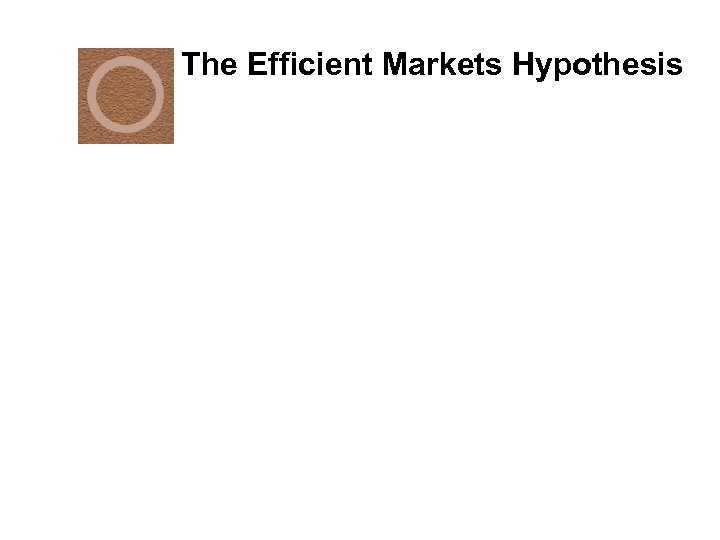The Efficient Markets Hypothesis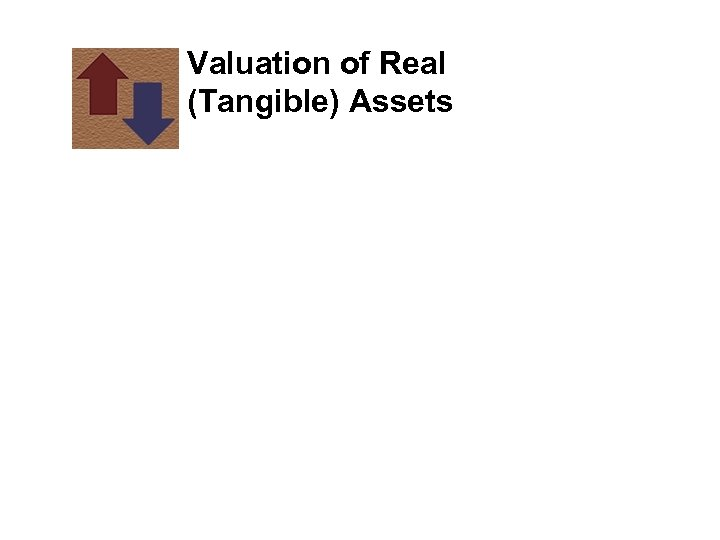Valuation of Real (Tangible) AssetsValuation of Real (Tangible) Assets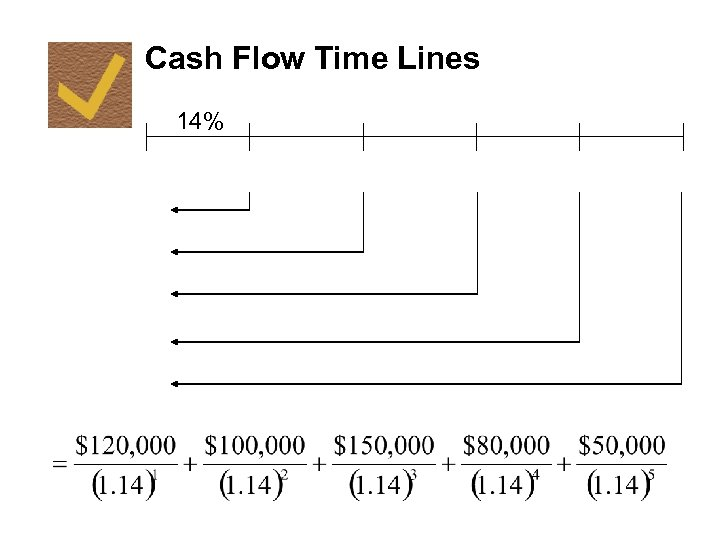Cash Flow Time Lines 14%